# What is Electric Current?

This page provides basic knowledge about electric current as well as an easy-to-understand introduction to topics like the difference between current and voltage, different types of current, and methods for measuring current. Once you’ve read it, you should have a basic understanding of electric current.

## What is electric current?

Electric current refers to the flow of electricity in an electronic circuit, and to the amount of electricity flowing through a circuit. It is measured in amperes (A). The larger the value in amperes, the more electricity is flowing in the circuit.

Electricity is easy to visualize if you think of it as the flow of water in a river (See Fig.1). Particles called electrons come together, and the number of electrons flowing each second is the current.

## Difference between voltage and current

Voltage is another term that’s used in regards to electronic circuits about as often as current. Voltage is measured in volts (V). Like current, voltage is also related to the flow of electrons in a circuit. Current refers to the flow of electrons, while voltage refers to the amount of force pushing the flowing electrons.

The higher the voltage, the more current will flow; a lower voltage means a weaker current.

Resistance also has a significant impact on current flow. Think of resistance as the width through which electrons flow. The greater the resistance, the narrower the width through which the electrons must flow, and therefore the lower the current. By contrast, a lower resistance increases the width through which electrons can flow, allowing more current to flow at once.

•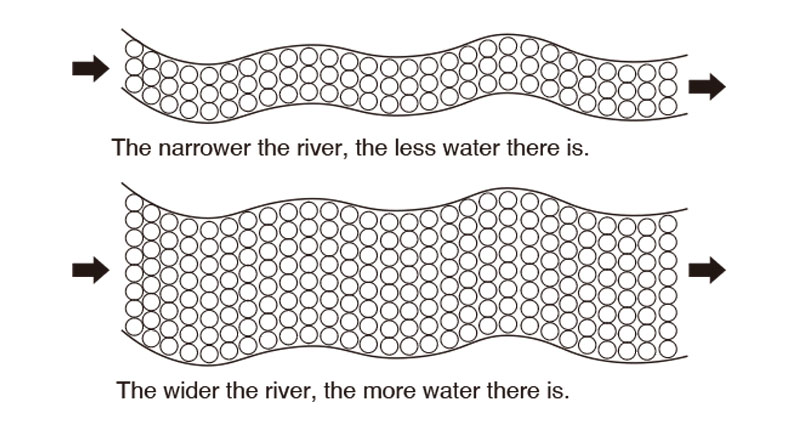Fig.1: Resistance and current can be compared to the flow of a river

If you want more current to flow at a given resistance value, you can accomplish that by raising the voltage. Power is generally calculated by multiplying current (A) by voltage (V), yielding a result that is expressed in watts (W). In this way, current and voltage are completely different, but both are important elements in the world of electricity.

## Direct current and alternating current

### Direct Current (DC)

The terms current and voltage encompass different types of phenomena, and one major distinction that can be made is that of direct current and alternating current. Direct current (DC) refers to current and voltage whose direction does not change.

A typical example is the electricity provided by dry cells and the lithium-ion batteries used in cars. With a direct current, the voltage is always positive (or always negative), and the current always flows in the same direction. As a result, a device may not operate if its battery is installed with the poles reversed.

•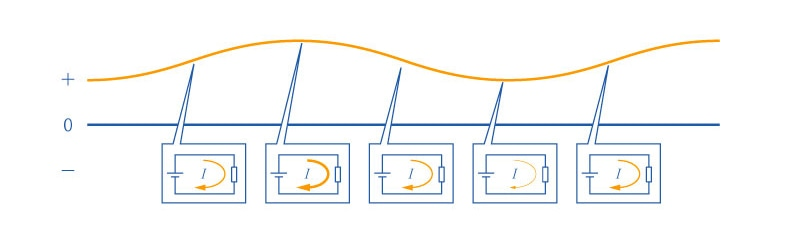### Alternating Current (AC)

By contrast, alternating current (AC) refers to current and voltage whose direction and magnitude vary regularly over time. AC current waveforms are distinguished by a variety of shapes, including sine waves, square waves, sawtooth waves, and triangular waves.

•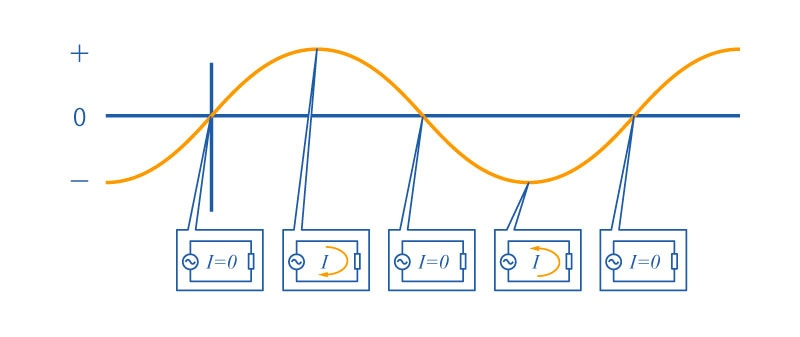AC electricity is used by the power grid, for example in household outlets. However, most standard electronic devices convert it into DC current with their internal circuitry. Why, then, does the power grid use AC current?

The reason has to do with transmission. Resistance in power lines causes losses when current is transmitted, but that loss can be reduced by increasing the voltage. However, it’s difficult to create high-voltage DC current, so electricity is transmitted as AC current and then stepped down to a lower voltage by transformers before being supplied to electric devices via the power grid. Then those devices, in most cases, convert the AC current into DC current with their internal circuitry so that it can be used.

## Methods for measuring electric current

To measure electric current, you’ll need to use an instrument such as a digital multimeter. Functionality varies by product, but digital multimeters can make a variety of measurements, including not only current, but also voltage and resistance.

When measuring electric current with a digital multimeter, you must set the instrument to its current function before making measurements. The instrument will have a number of display units, for example μA, mA, and A, so you’ll need to select the measurement range that best suits the current you’re measuring.

•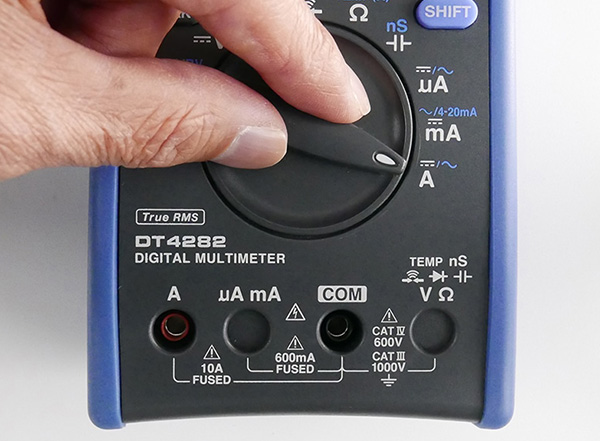When measuring current, connect the negative terminal to the COM receptacle and the positive terminal to the A receptacle on the instrument so that the multimeter is in series with the circuit.

•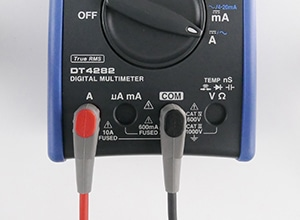•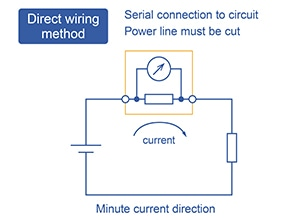### Precautions when measuring current

Exercise caution not to apply a voltage while the current function is selected. Doing so could damage the instrument by causing an overcurrent to flow through it. In fact, instruments use fuses to protect their circuitry, but it’s good practice to exercise caution since an overcurrent could damage the instrument. Some digital multimeters lack a current input terminal in order to avoid this hazard.

•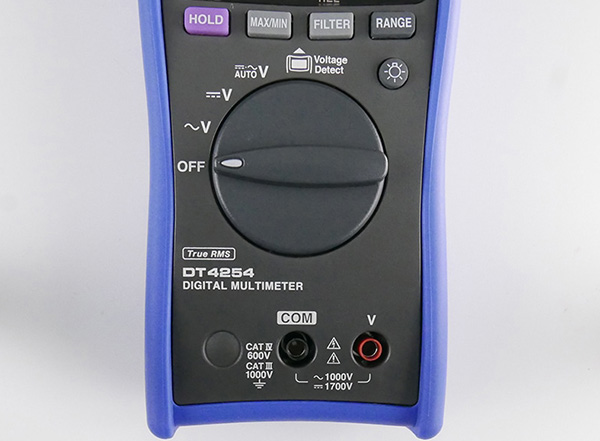### Using a digital multimeter to measure current

Current refers to the flow of electricity in an electronic circuit, with larger figures indicating more electricity. Although current differs from voltage, both are important concepts, and it's necessary to understand each.

Current can be measured using a digital multimeter. Why not try measuring current based on the information introduced in this page?